# RRB PO Mains Quantitative Aptitude (Day-01)

Quantitative Aptitude for RRB PO 2020

Dear Aspirants, Our IBPS Guide team is providing new series of Quantitative Aptitude Questions for RRB PO 2020 Mains so the aspirants can practice it on a daily basis. These questions are framed by our skilled experts after understanding your needs thoroughly. Aspirants can practice these new series questions daily to familiarize with the exact exam pattern and make your preparation effective.

Start Quiz

Caselet

Direction (1-5): Study the following information carefully and answer the questions given below.

A company has 4 different departments – IT, Sales, HR and Finance. The number of female employees from IT department is 800 and the ratio of the number of male to female employees from HR department is 3: 2. The number of female employees from IT department is 80 less than the number of male employees from Finance department. The number of female employees from IT department is 160 less than the number of male employees from HR department. Ratio of the number of male to female employees from Finance department is 2: 3 and the total number of male employees from all the departments together is 800 more than of the total number of female employees from all the departments together. The ratio of the number of male to female employees from IT department is 2: 1 and the number of female employees from Sales department is 480.

1) What is the difference between the total number of employees from Finance and HR department?

A) 400

B) 500

C) 600

D) 700

E) None of these

2) If 60% of the male employees from IT departments are transferred to Sales department and 55% of the female employees from sales department are transferred to IT department, then what is the ratio of the total number of employees from IT and Sales department together?

A) 67: 79

B) 69: 71

C) 71: 74

D) 73: 75

E) None of these

3) What is average number of employees from all the departments together?

A) 1640

B) 1820

C) 2050

D) 2280

E) None of these

4) If 20% of the number of male employees from Finance department is resign their jobs and then 20% of the female employees transferred to other department, then what is the total employees of the finance department?

A) 1680

B) 1730

C) 1760

D) 1790

E) None of these

5) What is the difference between total number of male and female employees from all the departments together?

A) 600

B) 700

C) 800

D) 500

E) None of these

Data Interpretation

Direction (6-10): Study the following information carefully and answer the questions given below.

The given pie graph shows the number of students in six different colleges.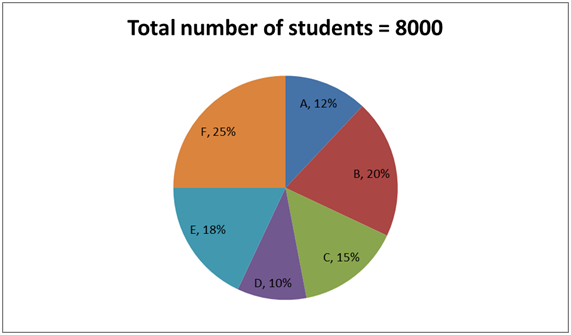Exam fee for boys and girls is Rs.80 and Rs.50 respectively.

The given table shows the ratio of boys and girls in different colleges.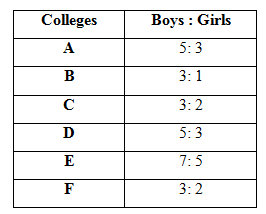6) What is the difference between the total exam fees collected from A and C?

A) Rs.13500

B) Rs.14800

C) Rs.15600

D) Rs.16200

E) None of these

7) Which of the following statements is correct according to the given information?

A) Total fee collected from boys in A is equal to Total fee collected from girls in D

B) Total fee collected from boys in B is equal to Total fee collected from girls in E

C) Total fee collected from boys in C is equal to Total fee collected from girls in B

D) Total fee collected from boys in F is equal to Total fee collected from girls in C

E) Total fee collected from boys in D is equal to Total fee collected from girls in F

8) What is the total number of boys from all the colleges together?

A) 8410

B) 5060

C) 8450

D) 5470

E) 5400

9) What is the difference between the total fees collected from boys and girls in all the colleges together?

A) Rs.227800

B) Rs.237800

C) Rs.247800

D) Rs.257800

E) None of these

10) Number of girls from E is what percent of the total number of students from B?

A) 32.5%

B) 35%

C) 37.5%

D) 42.5%

E) None of these

Direction (1-5):

Number of female employees from IT = 800

Number of male employees from IT = 2/1 * 800 = 1600

Number of male employees from Finance = 800 + 80 = 880

Number of female employees from Finance = 3/2 * 880 = 1320

Number of male employees from HR = 800 + 160 = 960

Number of female employees from HR = 2/3 * 960 = 640

Number of female employees from sales = 480

Total number of female employees = 800 + 1320 + 640 + 480 = 3240

Total number of male employees = 3240 + 800 = 4040

Number of male employees from Sales = 4040 – 1600 – 880 – 960 = 600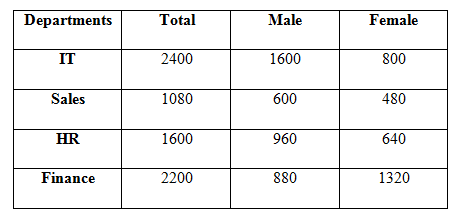Finance = 2200

HR = 1600

Difference = 2200 – 1600 = 600

60% of the male employees from IT department = 60/100 * 1600 = 960

Now the total male employees from IT department = 1600 * 40/100 = 640

Now the total number of male employees from sales department = 600 + 960

= 1560

55% of the female employees from sales department = 480 * 55/100 = 264

Now the female employees from sales department = 480 * 45/100 = 216

Total number of employees from sales department = 216 + 1560 = 1776

Total number of employees from IT department = 640 + 264 + 800 = 1704

Required ratio = 1704: 1776 = 213: 222

= 71: 74

Total number of employees from IT = 2400

Total number of employees from HR = 1600

Total number of employees from sales = 1080

Total number of employees from Finance = 2200

Average = (2400 + 2200 + 1080 + 1600)/4 = 1820

Number of male employees from finance = 880 * 80/100 = 704

Number of female employees from finance = 1320 * 80/100 = 1056

Total employees from finance

Total number of male employees = 1600 + 880 + 600 + 960 = 4040

Total number of female employees = 800 + 1320 + 480 + 640 = 3240

Difference = 4040 – 3240 = 800

= 704 + 1056 = 1760

Direction (6-10):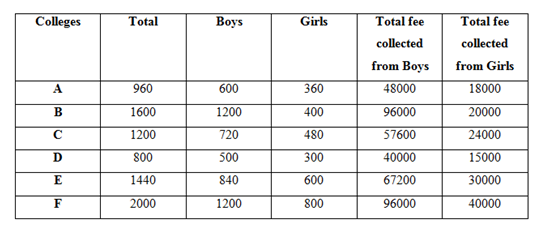Total collection from A = 48000 + 18000 = 66000

Total collection from C = 57600 + 24000 = 81600

Difference = 81600 – 66000 = 15600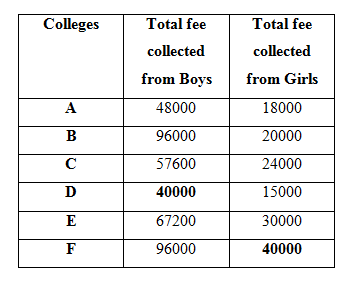Total number of boys = (600 + 1200 + 720 + 500 + 840 + 1200) = 5060

Total collection from boys = 48000 + 96000 + 57600 + 40000 + 67200 + 96000

= 404800

Total collection from girls = 18000 + 20000 + 24000 + 15000 + 30000 + 40000

= 147000

Difference = 404800 – 147000 = 257800